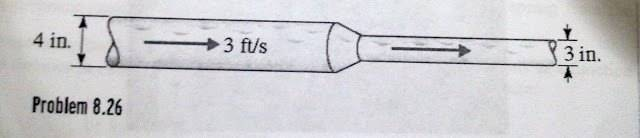# Speed of water flowing through pipe

## Homework Statement

The speed of water flowing through the "influent" 4-inch diameter section of the piping system below is 3.0 ft/s. What is the volume flow rate of water in the piping system? Express the volume flow rate in $ft^3/s$

## The Attempt at a Solution

I really don't know what to do. I know pi*r^2 comes into play here, but I need help. Just tell me how to start and guide me through it please.Flow rate = area of the pipe multiplied by the velocity.

You have everything but the flow rate. The area of the pipe is pi*r^2 as you said. Since you're not given a second density we can assume the flow is incompressible, and due to the conservation of mass, the flow rate in the 3 in dimater pipe will be the same. Q1 = Q2. I can't give you anymore without solving it for you.

SteamKing
Staff Emeritus
Homework Helper
Given that the fluid is water, it's safe to assume that the fluid is incompressible.

... the flow rate in the 3 in dimater pipe will be the same. Q1 = Q2.
Can the speed of the water be different even though the flow rate is the same? Part two says to find the speed of the water in the 3-inch diameter section in ft/s

SteamKing
Staff Emeritus
Homework Helper
Yes.

bigfooted
Gold Member
Can the speed of the water be different even though the flow rate is the same? Part two says to find the speed of the water in the 3-inch diameter section in ft/s

Yes, this is connected to the equation given by vadar2012: flow rate = area x velocity.
The flow rates are the same everywhere in the pipe (it must be, the water doesn't disappear). Therefore, if the area of the pipe increases, the velocity decreases.

So:
step 1: calculate flow rate = area x velocity or Q1=A1 x V1
step 2: use Q1 = Q2 to calculate V2